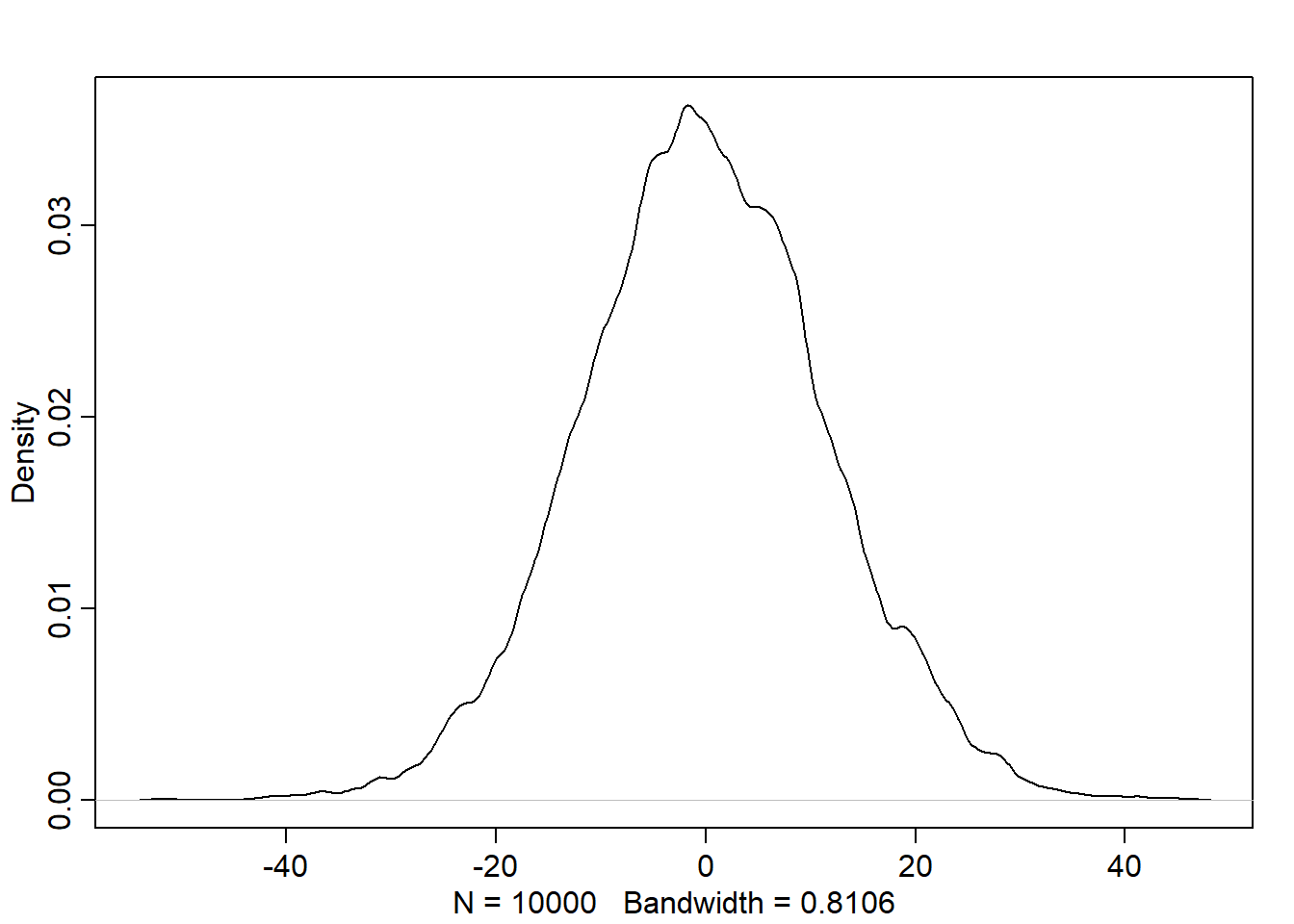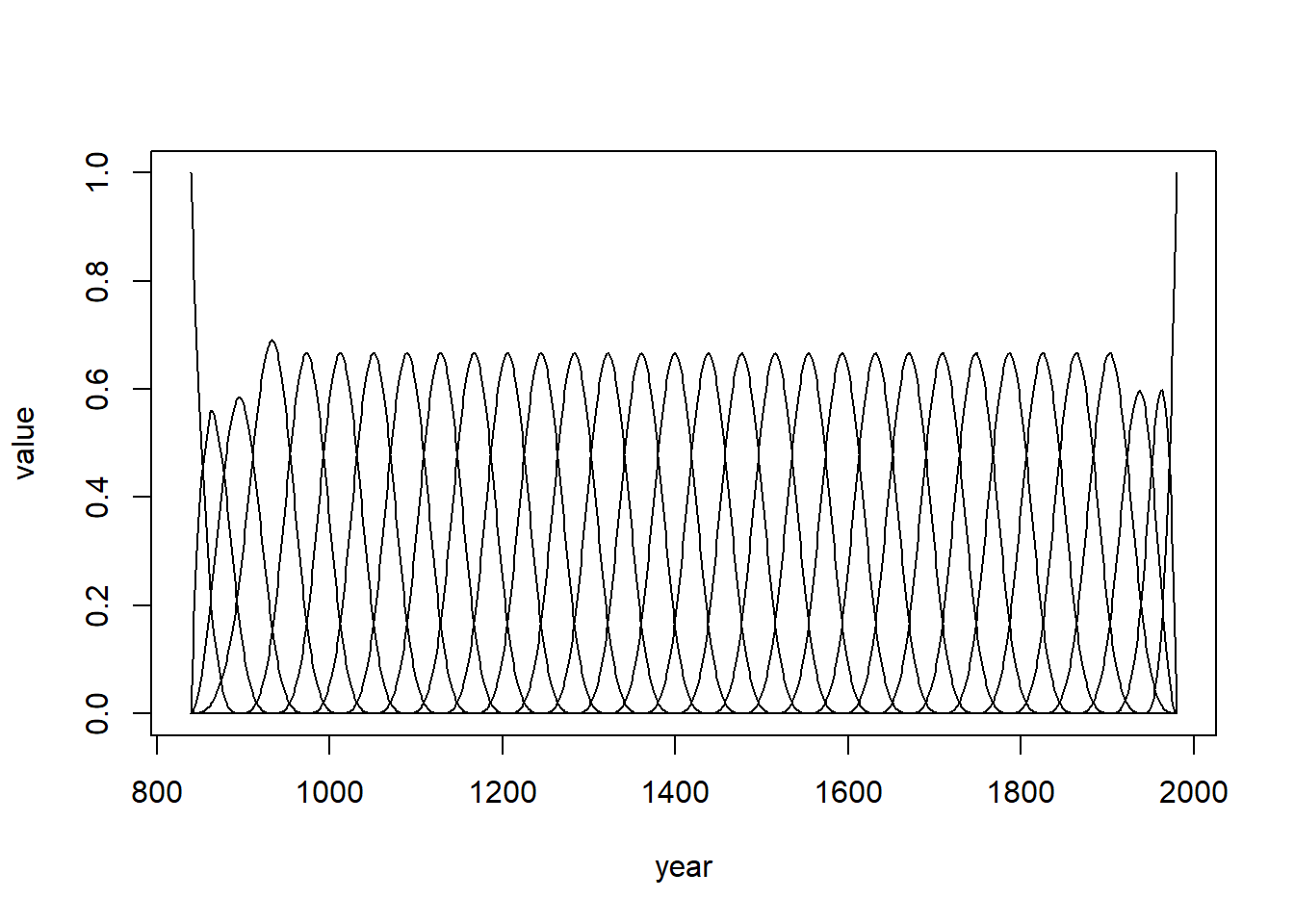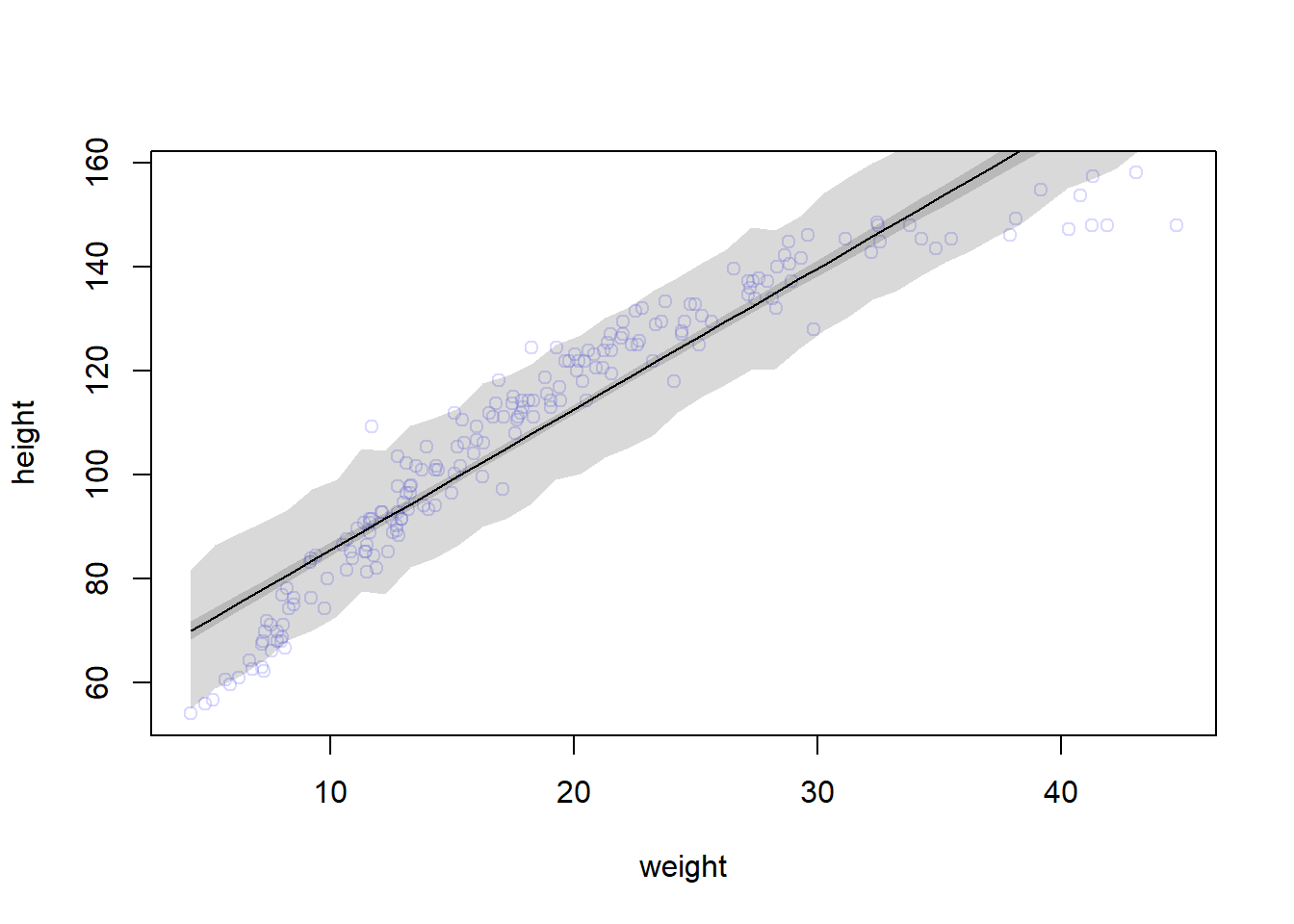## Chapter 4 - Geocentric Models

This chapter introduced the simple linear regression model, a framework for estimating the association between a predictor variable and an outcome variable. The Gaussian distribution comprises the likelihood in such models, because it counts up the relative numbers of ways different combinations of means and standard deviations can produce an observation. To fit these models to data, the chapter introduced quadratic approximation of the posterior distribution and the tool quap. It also introduced new procedures for visualizing prior and posterior distributions.

Place each answer inside the code chunk (grey box). The code chunks should contain a text response or a code that completes/answers the question or activity requested. Make sure to include plots if the question requests them. Problems are labeled Easy (E), Medium (M), and Hard(H).

Finally, upon completion, name your final output .html file as: Ronak jain_ANLY505-Year-Semester.html and publish the assignment to your R Pubs account and submit the link to Canvas. Each question is worth 5 points.

## Questions

4E1. In the model definition below, which line is the likelihood? \begin{align} \ y_i ∼ Normal(μ, σ) \\ \ μ ∼ Normal(0, 10) \\ \ σ ∼ Exponential(1) \\ \end{align}

#y_i ∼ Normal(μ, σ)

4E2. In the model definition just above, how many parameters are in the posterior distribution?

#Two: μ7, σ 

4E3. Using the model definition above, write down the appropriate form of Bayes’ theorem that includes the proper likelihood and priors.

# Pr(μ,σ|y) =(ΠiNormal(yi|μ, σ)Normal(μ|0,10)Uniform(σ|0,10))/ ∫∫ΠiNormal(hi|μ, σ)Normal(μ|0,10)Uniform(σ|0,10)dμdσ

4E4. In the model definition below, which line is the linear model? \begin{align} \ y_i ∼ Normal(μ, σ) \\ \ μ_i = α + βx_i \\ \ α ∼ Normal(0, 10) \\ \ β ∼ Normal(0, 1) \\ \ σ ∼ Exponential(2) \\ \end{align}

# μ_i = α + βx_i

4E5. In the model definition just above, how many parameters are in the posterior distribution?

# There are 3 parameters -  α, β, σ

4M1. For the model definition below, simulate observed y values from the prior (not the posterior). Make sure to plot the simulation. \begin{align} \ y_i ∼ Normal(μ, σ) \\ \ μ ∼ Normal(0, 10) \\ \ σ ∼ Exponential(1) \\ \end{align}

sample_mu <- rnorm(1e4, 0, 10)
sample_sigma <- runif(1e4, 0, 10)
prior_y <- rnorm(1e4, sample_mu, sample_sigma)
dens(prior_y)4M2. Translate the model just above into a quap formula.

formula <- alist(y ~ dnorm(mu, sigma),mu ~ dnorm(0, 10),sigma ~ dunif(0,10))
4M3. Translate the quap model formula below into a mathematical model definition:

y ~ dnorm( mu , sigma ),
mu <- a + b*x,
a ~ dnorm( 0 , 10 ),
b ~ dunif( 0 , 1 ),
sigma ~ dexp( 1 )

flist <- alist(y ~ dnorm(mu, sigma),mu <- a + b*x,a ~ dnorm(0, 50),
b ~ dunif(0, 10),  sigma ~ dunif(0, 50)
)

4M4. A sample of students is measured for height each year for 3 years. After the third year, you want to fit a linear regression predicting height using year as a predictor. Write down the mathematical model definition for this regression, using any variable names and priors you choose. Be prepared to defend your choice of priors. Simulate from the priors that you chose to see what the model expects before it sees the data. Do this by sampling from the priors. Then consider 50 students, each simulated with a different prior draw. For each student simulate 3 years. Plot the 50 linear relationships with height(cm) on the y-axis and year on the x-axis. What can we do to make these priors more likely?

# μ_i = α + βx_i
# σ ∼ Uniform(0,50)
# h_i ∼ Normal(μ, σ)
# α ∼ Normal(150, 25)
# β ∼ Normal(4, 2)

# I select a linear model. Selected normal distribution for alpha prior at 150 cm with standard deviation of 25cm. 150 cm is middle and i think 25cm is correct variance to include most students with high and low age distribution. Similarly selected a normal distribution for B prior with standard deviation of 2cm/years and mean at 4cm/year. And 2cm variance is good enough to include the students at low and high end age distribution. Selected uniform distribution for sigma prior i.e 0-50 cm as it this range includes both students at school and colleges.

4M5. Now suppose I remind you that every student got taller each year. Does this information lead you to change your choice of priors? How? Again, simulate from the priors and plot.

# No

4M6. Now suppose I tell you that the variance among heights for students of the same age is never more than 64cm. How does this lead you to revise your priors?

# As the variance cannot be more than square of 64 i.e 8. We can adjust the max of priors, but I am not confident to revise the priors.

4M7. Refit model m4.3 from the chapter, but omit the mean weight xbar this time. Compare the new model’s posterior to that of the original model. In particular, look at the covariance among the parameters. Show the pairs() plot. What is different? Then compare the posterior predictions of both models.

data("Howell1")
d <- Howell1
d2 <- d[ d$age >= 18, ] xbar <- mean( d2$weight )
m4.3 <- quap(
alist(
height ~ dnorm( mu, sigma),
mu <- a + b * ( weight - xbar ) ,
a ~ dnorm( 178, 20),
b ~ dlnorm( 0, 1),
sigma ~ dunif(0, 50)
) ,
data = d2
)
precis(m4.3)
##             mean         sd        5.5%       94.5%
## a     154.601363 0.27030771 154.1693587 155.0333666
## b       0.903281 0.04192364   0.8362789   0.9702831
## sigma   5.071882 0.19115486   4.7663794   5.3773842
m4.3_New <- quap(
alist(
height ~ dnorm( mu, sigma),
mu <- a + b * weight ,
a ~ dnorm( 178, 20),
b ~ dlnorm( 0, 1),
sigma ~ dunif(0, 50)
) ,
data = d2
)
precis(m4.3_New)
##              mean         sd        5.5%       94.5%
## a     114.5354543 1.89796277 111.5021432 117.5687653
## b       0.8907155 0.04176271   0.8239706   0.9574604
## sigma   5.0732938 0.19130320   4.7675544   5.3790333
vcov(m4.3_New)
##                  a             b         sigma
## a      3.602262674 -0.0784556166  0.0093776271
## b     -0.078455617  0.0017441240 -0.0002045985
## sigma  0.009377627 -0.0002045985  0.0365969131
#alpha is much lower compared to that in the prior model which shows a strong negative correlation between the two.

4M8. In the chapter, we used 15 knots with the cherry blossom spline. Increase the number of knots and observe what happens to the resulting spline. Then adjust also the width of the prior on the weights—change the standard deviation of the prior and watch what happens. What do you think the combination of knot number and the prior on the weights controls?

data(cherry_blossoms)
d <- cherry_blossoms
d2 <- d[ complete.cases(d$temp) , ] num_knots <- 30 knot_list <- quantile( d2$year , probs=seq(0,1,length.out=num_knots))
library(splines)
B <- bs(d2$year, knots=knot_list[-c(1,num_knots)] , degree=3 , intercept=TRUE ) plot( NULL , xlim=range(d2$year) , ylim=c(0,1) , xlab="year" , ylab="value" )
for ( i in 1:ncol(B) ) lines( d2$year , B[,i])4H2. Select out all the rows in the Howell1 data with ages below 18 years of age. If you do it right, you should end up with a new data frame with 192 rows in it. 1. Fit a linear regression to these data, using quap. Present and interpret the estimates. For every 10 units of increase in weight, how much taller does the model predict a child gets? 2. Plot the raw data, with height on the vertical axis and weight on the horizontal axis. Superimpose the MAP regression line and 89% interval for the mean. Also superimpose the 89% interval for predicted heights. 3. What aspects of the model fit concern you? Describe the kinds of assumptions you would change, if any, to improve the model. You don’t have to write any new code. Just explain what the model appears to be doing a bad job of, and what you hypothesize would be a better model. d2 <- Howell1[Howell1$age < 18, ]
nrow(d2)
##  192
#a)
formula <- alist(
height ~ dnorm(mu, sigma),
mu <- a + b * weight,
a ~ dnorm(110, 30),
b ~ dnorm(0, 10),
sigma ~ dunif(0, 60)
)
m <- map(formula, data = d2)
precis(m, corr = TRUE)
##            mean         sd      5.5%     94.5%
## a     58.345406 1.39587382 56.114530 60.576282
## b      2.715053 0.06824058  2.605991  2.824115
## sigma  8.438059 0.43067988  7.749750  9.126369
#b)
plot(height ~ weight, data = d2, col = col.alpha(rangi2, 0.3))

weight.seq <- seq(from = min(d2$weight), to = max(d2$weight), by = 1)
mu <- link(m, data = data.frame(weight = weight.seq))
mu.mean <- apply(mu, 2, mean)
mu.HPDI <- apply(mu, 2, HPDI, prob = 0.89)
lines(weight.seq, mu.mean)
shade(height.HPDI, weight.seq)#c)
# It looks like the model is not predicting the height as it underestimates the height of middling weights and overestimates both low (<10) and high (>30) weights. I think going with polynomial regression would be better.